# What is the conversion of a regular expression to finite Automata (NFA)?

A Regular Expression is a representation of Tokens. But, to recognize a token, it can need a token Recognizer, which is nothing but a Finite Automata (NFA). So, it can convert Regular Expression into NFA.

## Algorithm for the conversion of Regular Expression to NFA

Input − A Regular Expression R

Output − NFA accepting language denoted by R

Method

For ε, NFA isFor a NFA is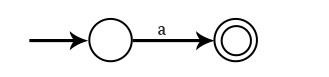For a + b, or a | b NFA is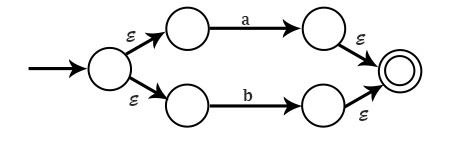For ab, NFA is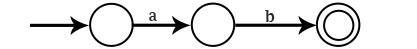For a*, NFA is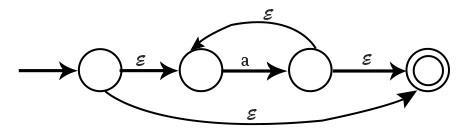Example1 − Draw NFA for the Regular Expression a(a+b)*ab

Solution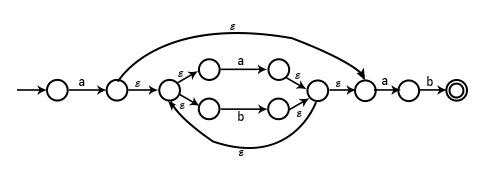Example2 − Draw NFA for a + b + ab

Solution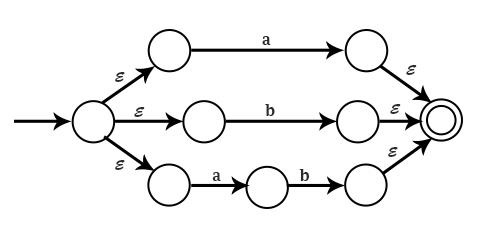Example3 − Draw NFA for letter (letter+digit)*

Solution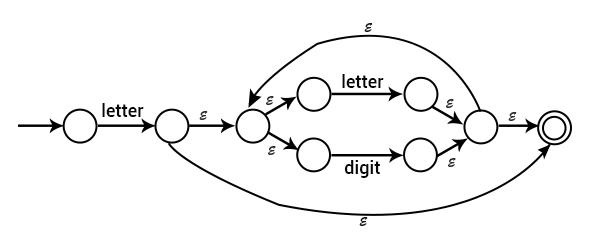Example4 − Draw NFA corresponding to (0+1)*1(0+1)

Solution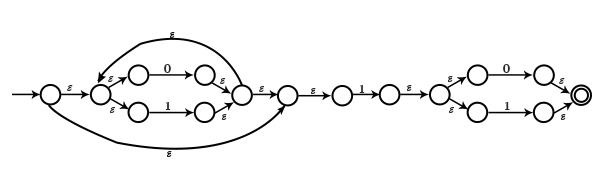ε−𝐜𝐥𝐨𝐬𝐮𝐫𝐞 (𝐬) − It is the set of states that can be reached form state s on ε−transitions alone.

• If s, t, u states. Initially, ε−closure (s)={s}.
• If s→t, then ε−closure (s)={s,t}.
• If s→t→u, then ε−closure (s)={s,t,u}

It will be repeated until all states are covered.

Algorithm: ε−𝐜𝐥𝐨𝐬𝐮𝐫𝐞 (𝐓)

T is a set of states whose ε−closure (s) is to be found.

Push All states in T on the stack

ε −closure (T)=T

While (stack not empty) {
Pop s, the top element of Stack
for each state t, with edge s→t {
if t is not present in ε−closure (T) {
ε−closure (T)=ε−closure (T)∪{t}
Push t on Stack
}
}
}

Example − Find ε−closure(0),ε−closure(1),ε−closure(4)for following NFA.

Solution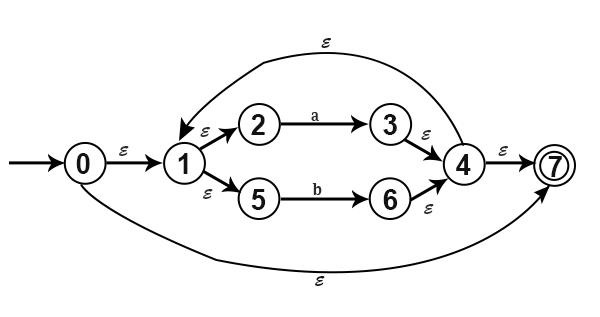ε−closure(0)={0,1,2,5,7}
ε−closure(1)={1,2,5}
ε−closure(4)={4,7,1,2,5}